##### 1. Introduction

The chief purpose of this blog is to introduce Machine Learning – one of the trendy and prevailing fields of computer science and some of its essential algorithms. The targeted audience of this blog should be varying from many fields, anyone who is interested in basic Machine Learning is warmly welcomed!

As depicted from the following image, as one of the most important subfields of AI, Machine Learning can also be translated as automatic learning, which is based upon the use of mathematical algorithms that intake input data and learn from it in order to solve a particular problem. The goal of Machine Learning is to recreate human interpretation behavior by implementing what it has learned from a problem and use that to solve similar issues.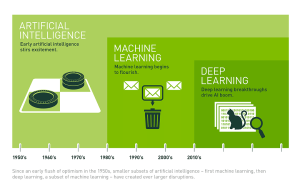Figure 1: Connection between AI, Machine Learning and Deep Learning

##### 2. Machine Learning Process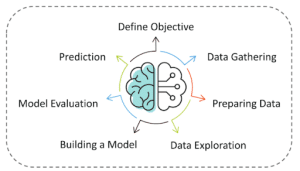Figure 2: Machine Learning Process

There are 7 major steps involved in the construction of a machine learning model to tackle a specific problem.

Define Objective: Understand what exactly needs to be predicted. It is also essential to take mental notes on what kind of data can be used to solve this problem or the type of approach you must follow to get to the solution

Data Gathering: Once you know the types of data that is required, you must understand how you can derive this data. Data collection can be done manually or by web scraping. You can always make use of open-source frameworks or platforms to help you retrieve data or you can take a quick search on the Internet and there should be plenty of resources suited for your requirement.

Data Preparation: The data you acquired is unlikely to be in the perfect format as there are lots of inconsistencies in data such as missing values, redundancies, duplicate variables, etc. Competent techniques to adjust or remove those variables is a must to avoid false computations during prediction or training

Data Exploration: This step is also known as Exploratory Data analysis (EDA) by using suitable visualization tools to dive deep into the data and explore the foreshadowed patterns. The outcome of this stage helps you to understand the data patterns/trends as well as draw out the correlations among the features.

Building a Model: This stage always begins by splitting the data set into two parts, training data, and testing data. The training data will be used to build and analyze the model. The logic of the model is based on the Machine Learning Algorithm that is being implemented.

Model Evaluation: After building a model by using the training data, the next step is to test out its competency and how accurately the model can get in the outcome

Prediction: Once the model is evaluated and improved, it is finally used to make predictions. The final output can be a Categorical variable (eg. True or False) or it can be a Continuous Quantity (eg. the predicted value of a stock).

##### 3. Types of Machine Learning
###### 3.1. By algorithms

Supervised Learning: Machine Learning model learns from labeled data. The purpose of this method is for the algorithm to be able to “learn” by comparing its actual output with the “taught” outputs to find errors and modify the model accordingly. Supervised learning therefore uses patterns to predict label values on additional unlabeled data.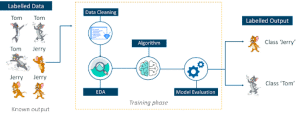Figure 3: Supervised Learning

Unsupervised Learning: Machine Learning model learns from unlabeled data, so it is forced to find the commonalities among the input data. Unlabeled data are more abundant than labeled data, therefore machine learning methods that facilitate unsupervised learning are particularly valuable. Besides revealing underlying patterns within the dataset, unsupervised learning is also utilized to perform feature learning, which helps computational machines disclose needed strategies to classify raw data.Figure 4: Unsupervised Learning

###### 3.2. By problems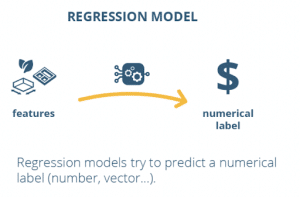Figure 5: Regression problem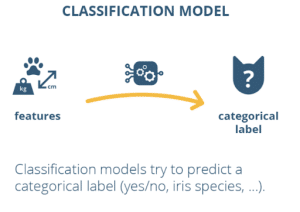Figure 6: Classification problem

Predicted labels can be either numbers or categories. For instance, we could be building a model that predicts market stock in a period, implying we would want to predict a label that’s a number. In this case, we would talk about a regression model.

Otherwise, we might also want to define a model that predicts a category, like “cat” or “not cat”, based on given features. In this situation, we would talk about a classification model.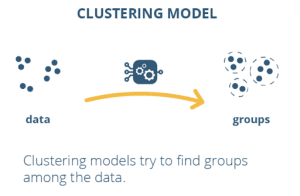Figure 7: Clustering problem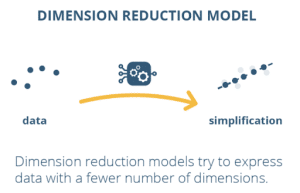Figure 8: Dimension reduction problem

With unsupervised learning, as we define a method to retrieve hidden structures only from features without any help from the label. If it tries to find groups among the data, we would talk about a clustering model. An example of a clustering model would be a model that segments different types of users in a social media platform.

Otherwise, if we have a model that transforms data and represents them with a smaller number of features, we would talk about a dimension reduction model. An example of this would be a model that summarizes the multiple technical characteristics of some cars into a few main indicators.

In summary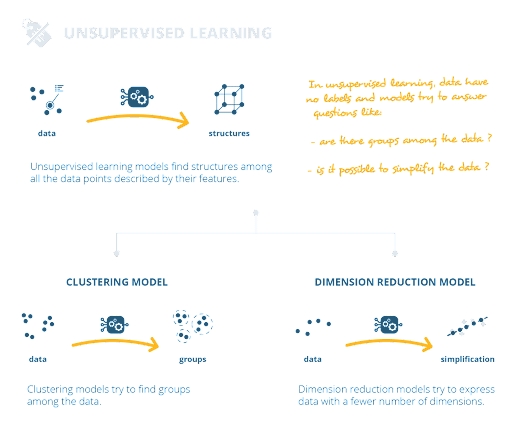Figure 9: Unsupervised learning wrap up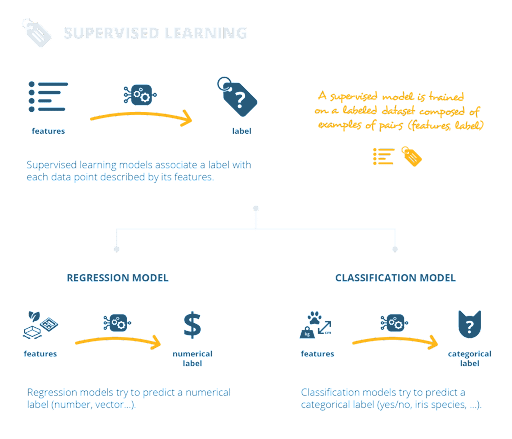Figure 10: Supervised wrap up

##### 4. Some conventional Machine Learning algorithms

For the sake of simplicity of this blog, some of the most commonly used Machine Learning algorithms in Supervised Learning are introduced.

###### 4.1. Linear Regression

It is a supervised learning algorithm, mainly used for predictive analysis. Linear regression makes predictions for continuous/real or numeric variables such as sales, salary, age, product price, etc.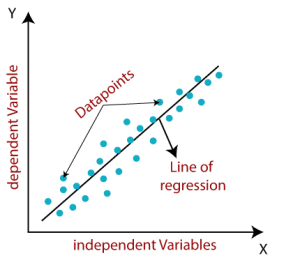Figure 11: What is Linear Regression?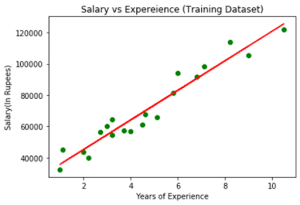Figure 12: Single Linear Regression

###### 4.2. Decision Tree

Another member from the supervised learning family, Decision Tree can be used for classification as well as regression problems. The name itself suggests that it uses a flowchart like a tree structure to show the predictions that result from a series of feature-based splits.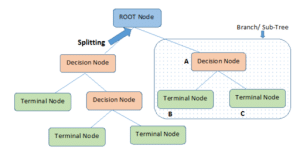Figure 13: What is Decision Tree algorithm?

Decision trees provide a way to present algorithms with conditional control statements. They include branches that represent decision-making steps that can lead to a favorable result.

There are 2 types of Decision Tree:

• Categorical variable: If the input is a categorical variable like whether the loan contender will defaulter or not, that is either yes/no. This type of decision tree is called a Categorical variable decision tree, also called classification trees.
• Continuous variable: If the input is numeric types and or is continuous in nature like when we need to predict a house price. Then the used decision tree is called a Continuous variable decision tree, also called Regression trees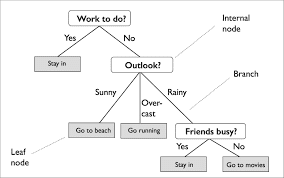Figure 14: Problem: Decision making on what kind of activities to do in a day?

###### 4.3. Random Forest

A random forest algorithm consists of many decision trees, in which each tree is slightly different from others. The (random forest) algorithm establishes the outcome based on the predictions of the decision trees. It predicts by taking the average or mean of the output from various trees.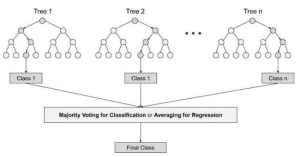Figure 15: What is Random Forest algorithm?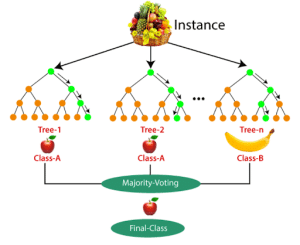Figure 16: Problem: Categorize a specific fruit in a basket containing various fruits

###### 4.4. Logistic Regression

It is used to calculate or predict the probability of a binary event occurring. For example, by using Logistic Regression, we can determine whether a particular mail is spam or not. The output between Linear and Logistic is different, one returns a numeric value while the other returns a probability whose value ranges between a define range (between 0 and 1).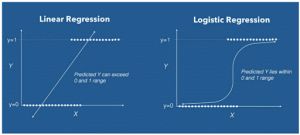Figure 17: Difference between Linear Regression and Logistic Regression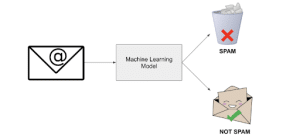Figure 18: Classify whether an email is spam or not using Logistic Regression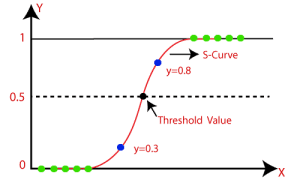Figure 19: How Logistic Regression works?

##### 5. Summary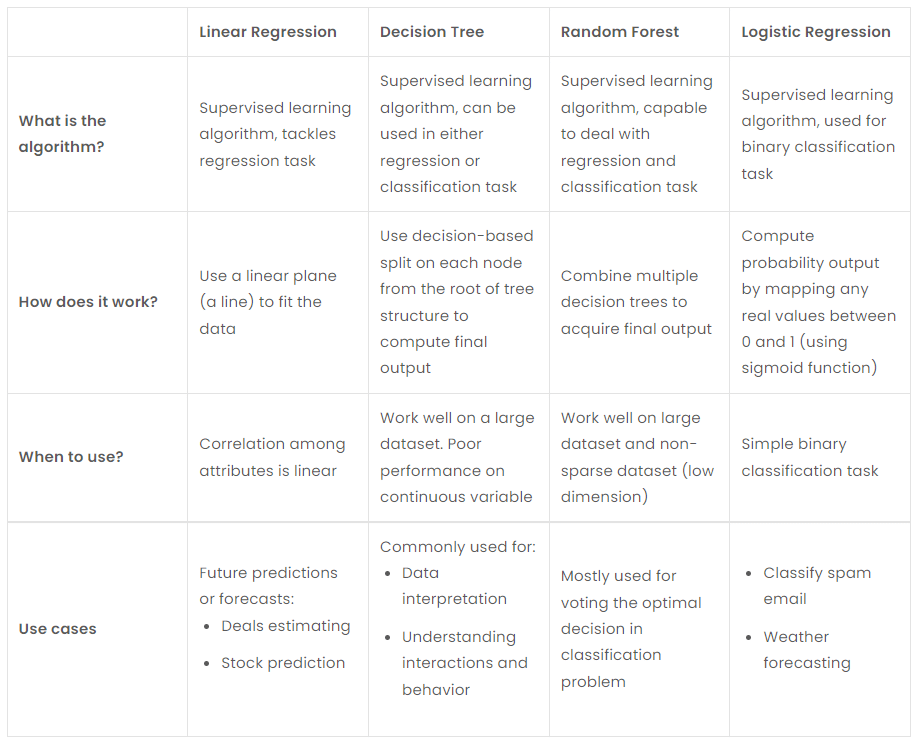——–

Machine Learning is one of the trendy and prevailing fields of computer science. Due to that, Mr. Triet Nguyen, member of the Data & AI team at Kyanon Digital, wants to share some useful information for everyone who is interested in it. We hope this blog will act as a starting guide for people from various professions to enter the field of Machine Learning.

ArchersTalk is a series of knowledge sharing events from members at Kyanon Digital (Archers) and also one of our 7 core values (Empowered Learning). Thanks to those sharing, not only other Archers but the external developers will also have the chance to get more useful knowledge and tips for better performance at work.

If you find these sharing useful, do not hesitate to share them with your friends and colleagues!

5/5 - (1 vote)